How to Create Table Calculations and Percentages in Tableau - dummies

# How to Create Table Calculations and Percentages in Tableau

Tableau offers a couple of different ways to add table calculations and percentages to the view. In most cases, you’ll probably choose to use the very convenient quick table calculations to look at things like running total, percent of total, and year-over-year growth.

However, we’ll take a different approach to creating a table calculation in order to show you all your options.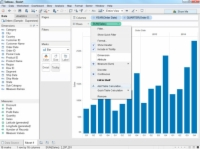## 1Build your view so that it contains a measure. Click the down arrow on the measure.

Doing so displays the Table Calculation dialog box. You could use the Quick Table Calculation in many cases, but this example shows the steps you’d need to carry out in order to add the calculations and define how it’s being computed.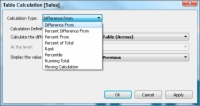## 3Choose the type of calculation you want.

Options are listed in the Calculation Type list box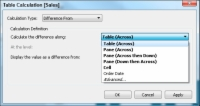## 4Select the additional options to define the calculation.

The available options differ depending on the type of calculation you’re adding to the view.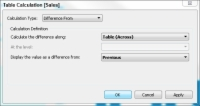You have completed the process, but you have a few things to check to make sure everything appears as it should.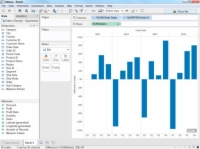## 6Check out the view after the table calculation has been added.

This is how the view appears after the table calculation has been added. Notice that Tableau indicates that the visualization contains a null value (the indicators in the lower-right corner of the view). This null value is the result of using a table calculation that shows the difference from the previous value. Because the first value in the view doesn’t have a previous value to compare with, the result is null, as expected.

When you add a table calculation, the dimensions in the table that define the section on the table that you’re computing along are called addressing fields, and the dimensions that define how to group the calculation are called partitioning fields. In this example, the category/region fields span the table, so they are considered addressing fields.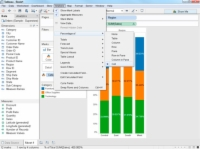## 7Choose any quick table calculations that you want to use.

It’s usually easier to choose one of the quick table calculations using the default values rather than trying to select all the options for table calculations using the Table Calculation dialog box.

Percentages are a specialized type of table calculation that shows the analysis as percentages rather than numeric values. Take a look at the options that appear when you choose Analysis → Percentage Of from Tableau’s main menu.

If you make a selection of a different type of table calculation such as a percentage, your existing table calculation is replaced. If you want to keep both types of views, make sure that you use the Duplicate Sheet button on the main toolbar to make a copy of the worksheet where you can choose a new type of calculation for the view.